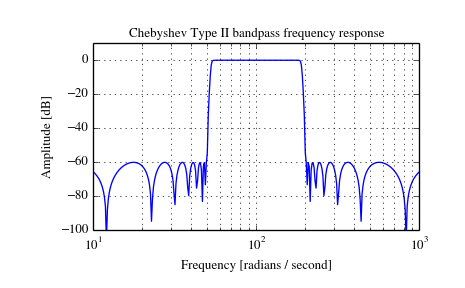# scipy.signal.iirfilter¶

scipy.signal.iirfilter(N, Wn, rp=None, rs=None, btype='band', analog=False, ftype='butter', output='ba')[source]

IIR digital and analog filter design given order and critical points.

Design an Nth order digital or analog filter and return the filter coefficients in (B,A) (numerator, denominator) or (Z,P,K) form.

Parameters: N : int The order of the filter. Wn : array_like A scalar or length-2 sequence giving the critical frequencies. For digital filters, Wn is normalized from 0 to 1, where 1 is the Nyquist frequency, pi radians/sample. (Wn is thus in half-cycles / sample.) For analog filters, Wn is an angular frequency (e.g. rad/s). rp : float, optional For Chebyshev and elliptic filters, provides the maximum ripple in the passband. (dB) rs : float, optional For Chebyshev and elliptic filters, provides the minimum attenuation in the stop band. (dB) btype : {‘bandpass’, ‘lowpass’, ‘highpass’, ‘bandstop’}, optional The type of filter. Default is ‘bandpass’. analog : bool, optional When True, return an analog filter, otherwise a digital filter is returned. ftype : str, optional The type of IIR filter to design: Butterworth : ‘butter’ Chebyshev I : ‘cheby1’ Chebyshev II : ‘cheby2’ Cauer/elliptic: ‘ellip’ Bessel/Thomson: ‘bessel’ output : {‘ba’, ‘zpk’}, optional Type of output: numerator/denominator (‘ba’) or pole-zero (‘zpk’). Default is ‘ba’.

butter
Filter design using order and critical points
buttord
Find order and critical points from passband and stopband spec
iirdesign
General filter design using passband and stopband spec

Examples

Generate a 17th-order Chebyshev II bandpass filter and plot the frequency response:

```>>> from scipy import signal
>>> import matplotlib.pyplot as plt
```
```>>> b, a = signal.iirfilter(17, [50, 200], rs=60, btype='band',
...                         analog=True, ftype='cheby2')
>>> w, h = signal.freqs(b, a, 1000)
>>> fig = plt.figure()
>>> ax.plot(w, 20 * np.log10(abs(h)))
>>> ax.set_xscale('log')
>>> ax.set_title('Chebyshev Type II bandpass frequency response')
>>> ax.set_ylabel('Amplitude [dB]')
>>> ax.axis((10, 1000, -100, 10))
>>> ax.grid(which='both', axis='both')
>>> plt.show()
```#### Previous topic

scipy.signal.iirdesign

#### Next topic

scipy.signal.kaiser_atten